# gggibbous

Moon charts, a pie chart alternative for two groups in ggplot2

## Installation

`gggibbous` can be installed from CRAN:

``install.packages("gggibbous")``

The development version can be installed from GitHub with the `devtools` package:

``devtools::install_github("mnbram/gggibbous")``

## Moon charts and pie charts

A pie chart divides a circle into multiple sections where the arc lengths (and so also the areas) of the sections represent proportions of a whole. A moon chart, similarly, divides a circle into sections where the areas represent proportions of a whole, but in a moon chart the areas are drawn as crescent or gibbous portions of a circle—like the phases of the moon.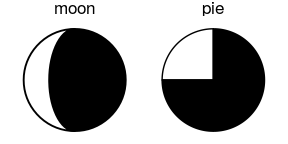The motivation behind using a moon chart instead of a pie chart is primarily one of aesthetic choice. Note also that because the sections of a moon chart are swept from one or the other side of the circle, they are generally only appropriate for depicting one or two groups.

Moon charts are similar to Kosara’s (2019) “circular slice” chart. In studying subjects’ perception of percentages in different chart types, the “circular slice” performed similarly to pie charts. Moon charts differ from the “circular slice” in that the latter slides a second disc of the same size over a base circle, more like a lunar eclipse than the sphases of the moon. Both depend on area as the perceptual cue, however.

## `gggibbous` and its usage

`gggibbous` extends the `ggplot2` data visualization package to provide support for moon charts in R. Unlike the pie charts supported natively by `coord_polar()` in R, moon charts in `gggibbous` do not require any special coordinate system. They are drawn most similarly to points in `ggplot2`: their position is defined by an `x` and a `y` coordinate and their size is defined independently of the coordinate system, so they always remain circular.

``````library(gggibbous)
``````ggplot(data.frame(x = 1:5, y = 1, size = 2^(0:4)), aes(x, y, size = size)) +
geom_moon() +
geom_point(y = 2) +
lims(x = c(0.5, 5.5), y = c(0.5, 2.5)) +
scale_size(range = c(5, 10))``````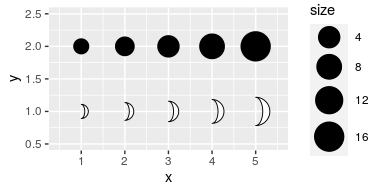Two new aesthetics are also important in `geom_moon`: `ratio` and `right`.

### The `ratio` aesthetic

`ratio` controls the proportion of the moon to be drawn. It must be between 0 (a “new moon” where nothing is actually drawn) and 1 (a “full moon”, i.e. a circle).

``````ggplot(data.frame(x = 1:5, y = 0, ratio = 0:4 * 0.25), aes(x = x, y = y)) +
geom_moon(aes(ratio = ratio), size = 20, fill = "black") +
geom_text(aes(y = y + 1, label = ratio)) +
lims(x = c(0.5, 5.5), y = c(-1, 1.4)) +
theme_void()``````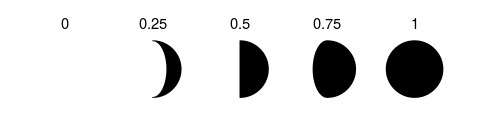### The `right` aesthetic

`right` takes a boolean value that controls whether the moon is “waxing” or “waning”—that is, whether it is “filled” from the right or the left.

One way to make a “complete” moon with two colors is to use `right = TRUE` for one color and `right = FALSE` for the other, with complementary ratios. (See the examples below for some other approaches.)

``````tidymoons <- data.frame(
x = rep(1:3, 6),
y = rep(rep(3:1, each = 3), 2),
ratio = c(1:9 / 10, 9:1 / 10),
right = rep(c(TRUE, FALSE), each = 9)
)

ggplot(tidymoons) +
geom_moon(aes(x, y, ratio = ratio, right = right, fill = right)) +
lims(x = c(0.5, 3.5), y = c(0.5, 3.5))``````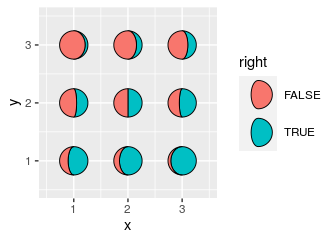## Legend key glyphs

`gggibbous` includes three key glyphs for different types of legends: `draw_key_moon`, `draw_key_moon_left`, and `draw_key_full_moon`

`draw_key_moon`—the default in `geom_moon`—draws a gibbous moon with `right = TRUE` (see above).

`draw_key_moon_left` draws a crescent moon from the left that is complementary to the gibbous moon in `draw_key_moon`, which is useful for combined legends:

``````ggplot(tidymoons, aes(x, y, ratio = ratio, right = right, size = 2^x)) +
geom_moon(data = subset(tidymoons, right), fill = "violetred") +
geom_moon(
data = subset(tidymoons, !right), fill = "turquoise3",
key_glyph = draw_key_moon_left
) +
lims(x = c(0.5, 3.5), y = c(0.5, 3.5)) +
scale_size("size", range = c(5, 10), breaks = 2^(1:3))``````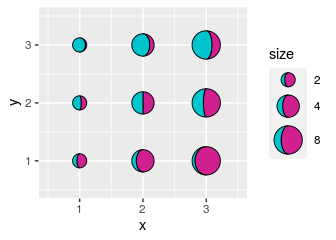`draw_key_full_moon` draws a circle. It is similar to the “point” key glyph, but the size is calculated slightly differently, so it is more appropriate if you want the size of legend moons and the size of the plot moons to match.

``````ggplot(tidymoons) +
geom_moon(
aes(x, y, ratio = ratio, right = right, fill = right, size = 2^x),
key_glyph = draw_key_full_moon
) +
lims(x = c(0.5, 3.5), y = c(0.5, 3.5)) +
scale_size("size", range = c(5, 10), breaks = 2^(1:3)) +
scale_fill_manual(values = c("firebrick1", "dodgerblue2")) +
theme(legend.box = "horizontal")``````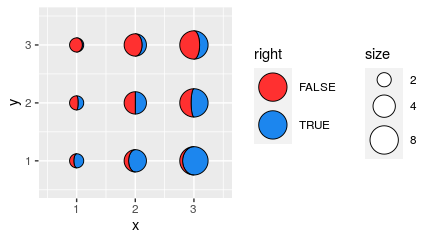## Worked examples

### Moon charts on maps

One common use of multiple pie charts is to represent proportions at different coordinates on a map. The x and y dimensions are already committed to the map coordinates, so proportional visualizations like bar charts are more difficult. This is a perfect opportunity to try out moon charts!

Pie chart maps are popular in population genetics, so let’s look at an example from that field. The `dmeladh` data contains frequencies of two variants of the Adh gene in Australian and Papua New Guinean fruit fly populations. Many of these populations are close together, so we have to deal with overplotting, which we do manually below.

``````dmeladh_adj <- dmeladh
-2, 0, -2, 2, -3, 3, 3, 2, 3, 4, -2.5, -2.5, -1, -2, -2.5, -4, 2.5,
5, 6, 7, 2, -7, -5.5, -3, 0, -7, -2, 3, 5.5, 0.5, -1, -1.5, -3, 2)
-2, 2, 0, 1, 0, 0, 0, 2, 0.5, -1, 1, -1.5, 2, 4, 1.5, 0, 2,
1, -1, -3, -2, 1, -1, -2, -3, -2, -4, -3, -1, 1.5, 2, 2, -2, 0)

geom_polygon(
data = map_data(
"world", region = "(Australia)|(Indonesia)|(Papua New Guinea)"),
aes(group = group),
fill = "gray80"
) +
geom_segment(aes(xend = Longitude, yend = Latitude), color = "gray20") +
geom_point(aes(Longitude, Latitude), size = 0.75, color = "gray20") +
scale_size(range = c(4, 10)) +
coord_map(xlim = c(110, 160), ylim = c(-45, -5)) +
theme_void() +
theme(
legend.position = c(0.05, 0.05),
legend.direction = "horizontal",
legend.justification = c(0, 0)
)

moonmap +
geom_moon(
aes(ratio = AdhS / 100, size = N),
right = FALSE, fill = "gold", color = "gold",
key_glyph = draw_key_moon_left
) +
geom_moon(
aes(ratio = AdhF / 100, size = N),
fill = "forestgreen", color = "forestgreen"
)``````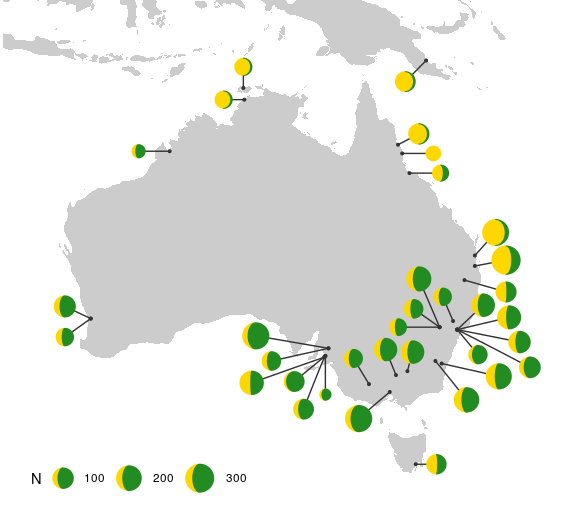If we want to label the alleles in the legend explicitly, then we need to map them to a group, which requires that we rearrange the data into a “longer” (“tidy”) format.

``````tidyadh <- reshape(
v.names = "percent",
timevar = "allele",
idvar = c("Locality", "Latitude", "Longitude", "long", "lat", "N"),
direction = "long"
)

moonmap +
geom_moon(
data = tidyadh, key_glyph = draw_key_full_moon,
aes(ratio = percent / 100, fill = allele, color = allele, right = right,
size = N)
) +
scale_fill_manual(values = c("forestgreen", "gold")) +
scale_color_manual(values = c("forestgreen", "gold"))``````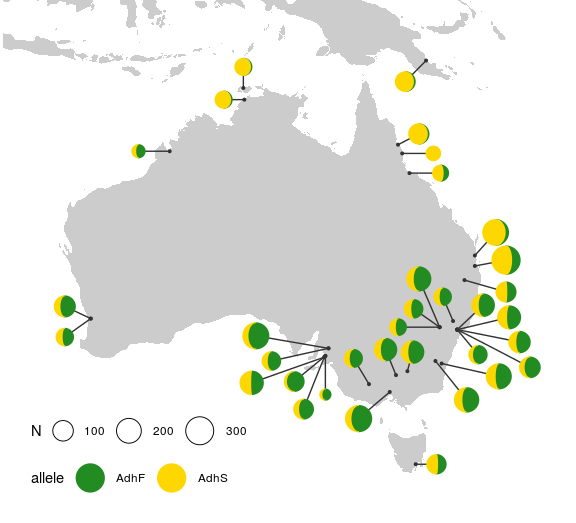### Lunar data

Sometimes you just want to plot literal representations of the moon. The `lunardist` data, adapted from NASA, contains the distance from the Earth to the Moon for each day in 2019, as well as the dates (in UTC) of each occurrence of the four principal phases of the moon. We can plot those principal phases using moon charts (which in this case are identical to pie charts).

``````moonphase <- subset(lunardist, !is.na(phase))
moonphase\$percent <- ifelse(
moonphase\$phase == "new", 0, ifelse(moonphase\$phase == "full", 1, 0.5))

ggplot(lunardist, aes(date, distance)) +
geom_line() +
# Plotting the lower layer as a full circle also works in most cases
geom_moon(data = moonphase, ratio = 1, size = 5, fill = "black") +
geom_moon(
data = moonphase, aes(ratio = percent),
size = 5, fill = "yellow", right = moonphase\$phase == "first quarter"
)``````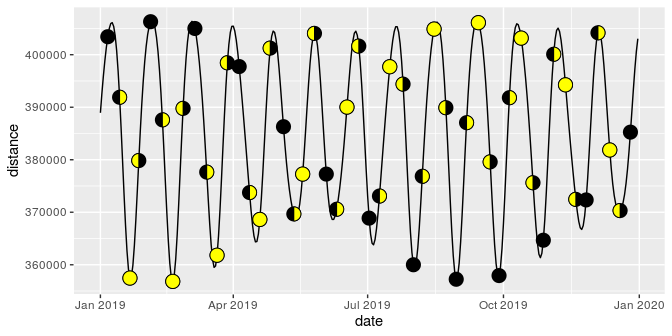### Harvey balls

“Harvey balls” are essentially pie charts used for qualitative comparisons, often in tabular format. We can use moon charts for the same purpose.

First, let’s make up some data:

``````rest_names <- c(
"Anscombe's Luncheonette", "Chai Squared", "Tukey's Honest Southern Diner",
"Bagels ANOVA", "Spearmint Row"
)
restaurants <- data.frame(
Restaurant = factor(rest_names, levels = rest_names),
Food = c(5, 3, 4, 4, 1),
Decor = c(2, 5, 3, 1, 5),
Service = c(4, 2, 3, 3, 5),
Price = c(4, 5, 2, 5, 2)
)``````

As a regular table:

Restaurant Food Decor Service Price
Anscombe’s Luncheonette 5 2 4 4
Chai Squared 3 5 2 5
Tukey’s Honest Southern Diner 4 3 3 2
Bagels ANOVA 4 1 3 5
Spearmint Row 1 5 5 2

And now as a table with Harvey moons:

``````# First we reshape the data into "long" format to facilitate plotting
rest_cats <- c("Food", "Decor", "Service", "Price")
tidyrest <- reshape(
restaurants,
varying = rest_cats,
v.names = "Score",
timevar = "Category",
times = factor(rest_cats, levels = rest_cats),
idvar = "Restaurant",
direction = "long"
)

ggplot(tidyrest, aes(0, 0)) +
geom_moon(aes(ratio = (Score - 1) / 4), fill = "black") +
geom_moon(aes(ratio = 1 - (Score - 1) / 4), right = FALSE) +
facet_grid(Restaurant ~ Category, switch = "y") +
theme_minimal() +
theme(
panel.grid = element_blank(),
strip.text.y.left = element_text(angle = 0, hjust = 1),
axis.text = element_blank(),
axis.title = element_blank()
)``````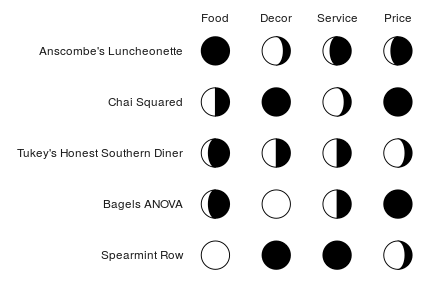1. Kosara, R. 2019. Circular Part-to-Whole Charts Using the Area Visual Cue. EuroVis 2019 - Short Papers. https://doi.org/10.2312/evs.20191163

2. Oakeshott, J.G., et al. 1982. Alcohol dehydrogenase and glycerol-3-phosphate dehydrogenase clines in Drosophila melanogaster on different continents. Evolution, 36(1): 86-96.